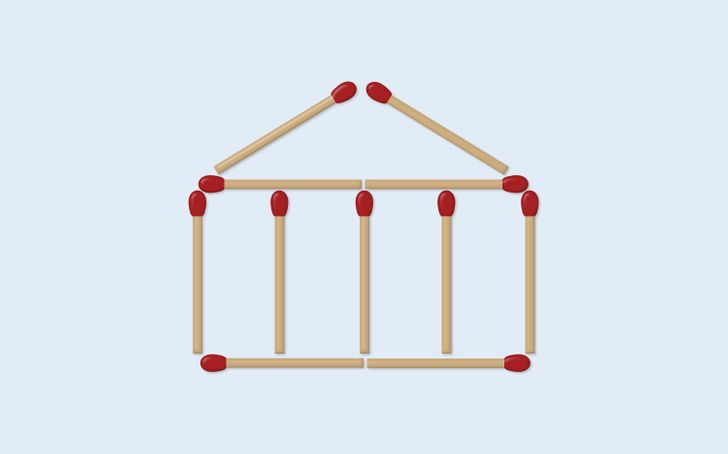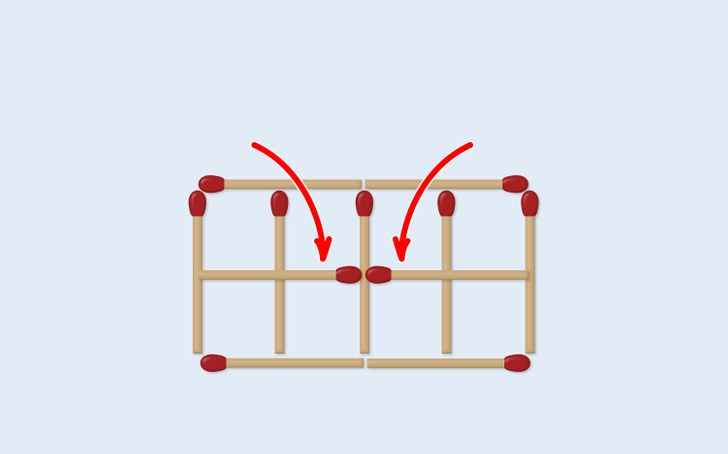# 16 Matchstick Puzzles to Fire Up Your Brain

Quizzes
2 years ago

Not all of us are mathematical geniuses and that is ok. But every once in a while, it is good to give your brain a nudge and put it to work. This will increase your focus and attention to detail. Take a piece of paper and write down your answers. You can also go to the kitchen, get some matches (don’t light them), and arrange them on a surface, like a table or a bed.

Bright Side gathered 16 matchstick puzzles for you that will definitely get your brain in gear. You can find out the answers by tapping on the photos. But control your urge and don’t sneak a peek before you try to figure them out.

## 1. Move one single matchstick to solve the mathematical equation below.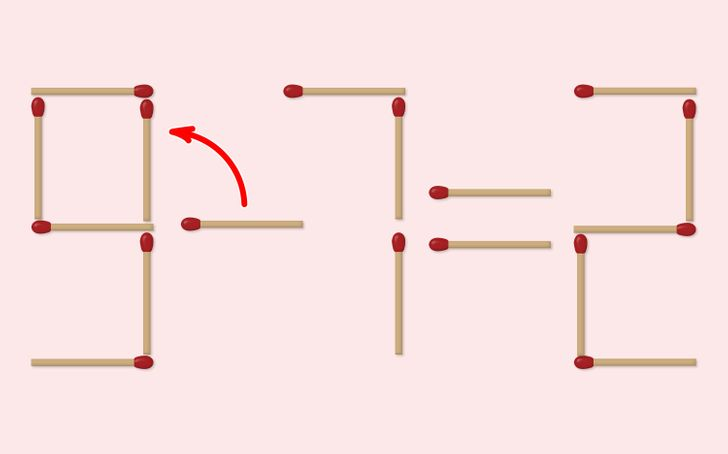## 2. Move only one match so that the equation becomes correct.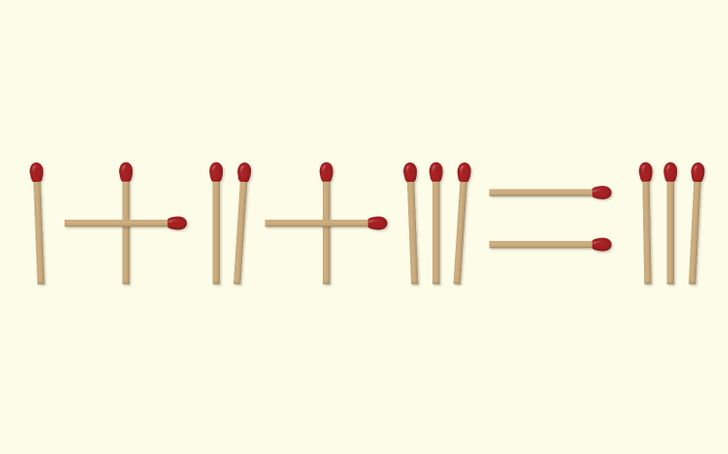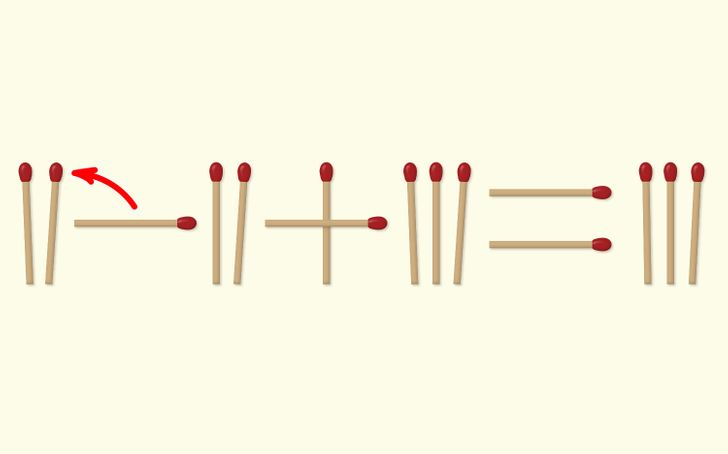## 3. You have 4 matches arranged like this. Move only one match to form a square.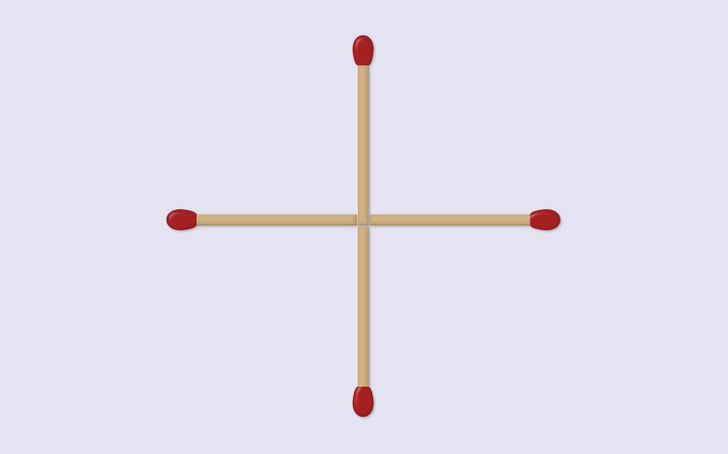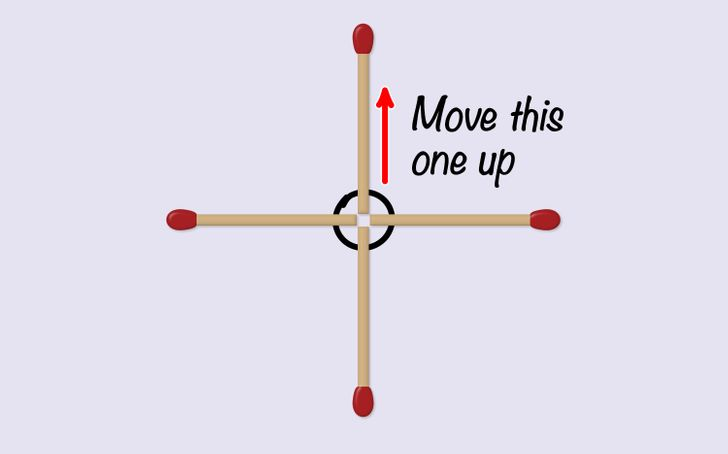## 4. Move only 2 matches so that the equation is correct.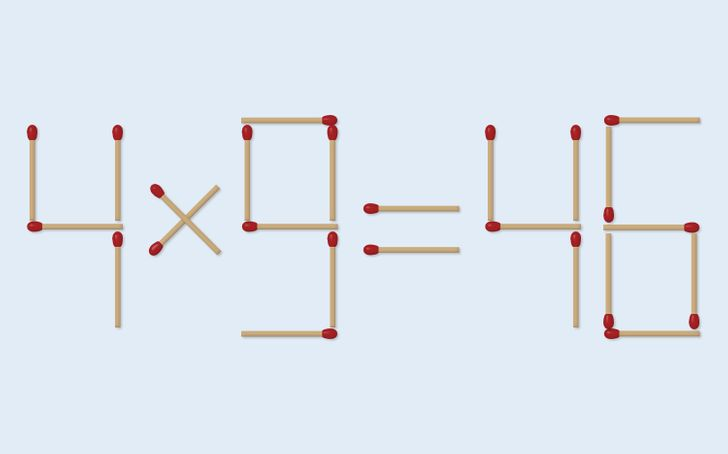## 5. Move 4 matches only. You need to form 2 smaller arrows.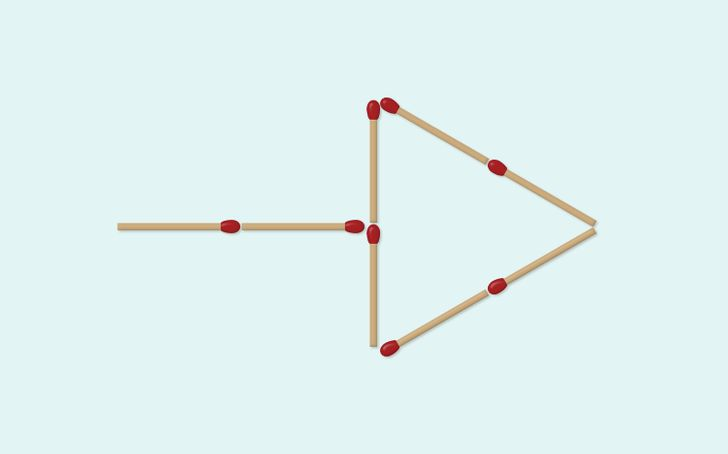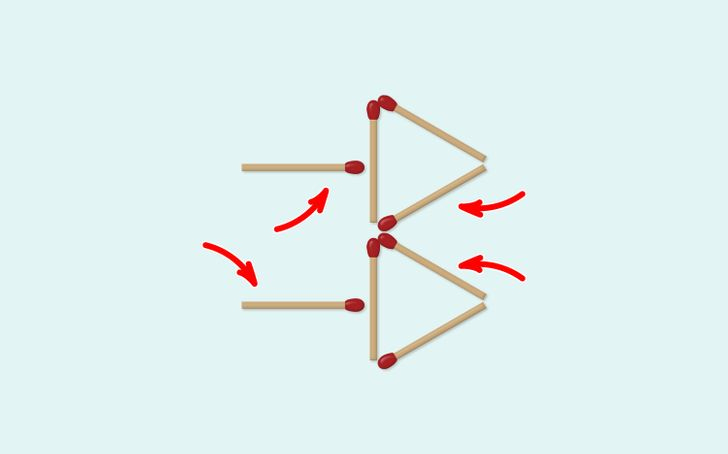## 6. Move only 3 matches and form 3 squares.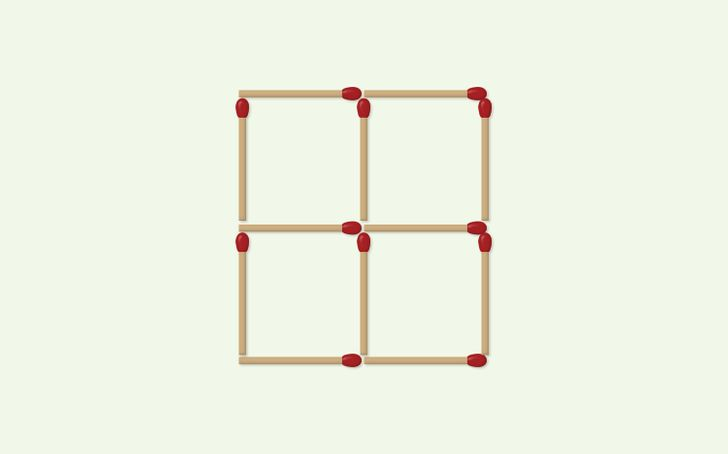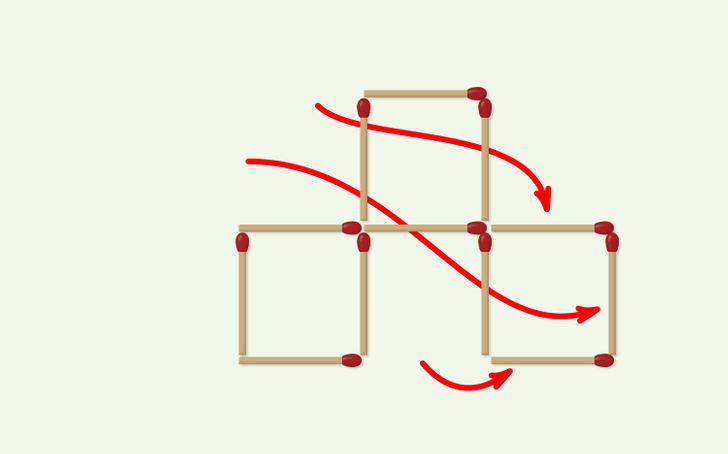## 7. Move 3 matchsticks to make 3 equal squares.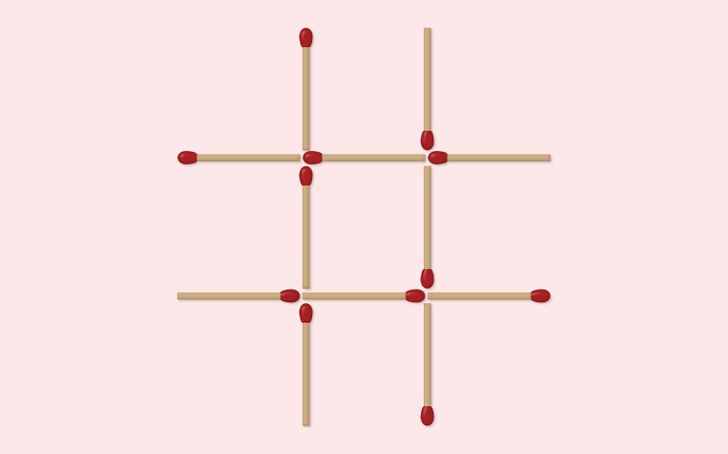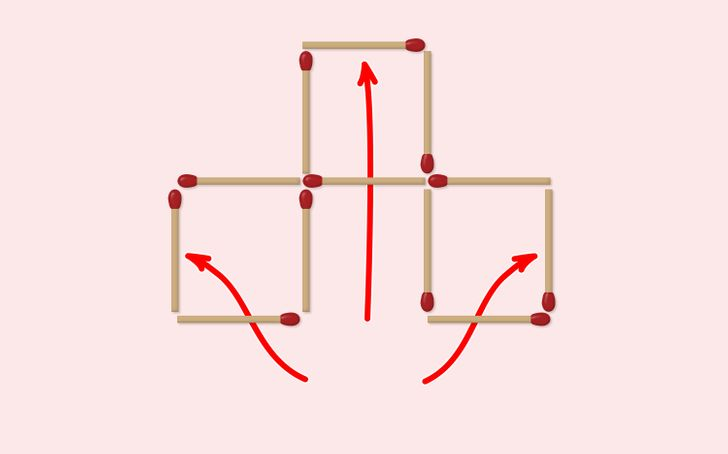## 8. Move only 3 matches so that the fish swims to the right.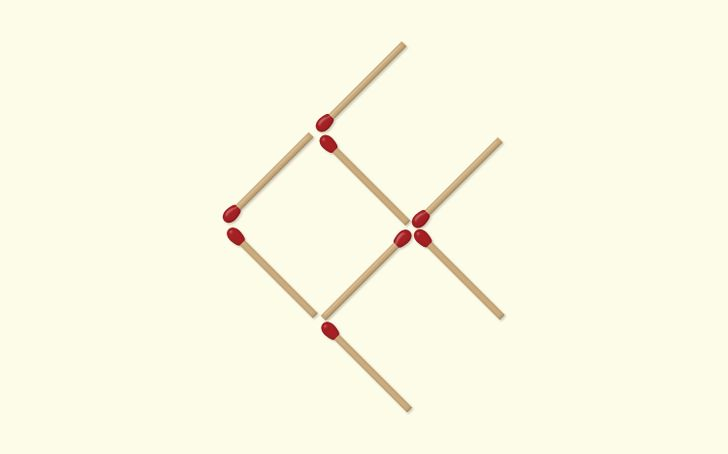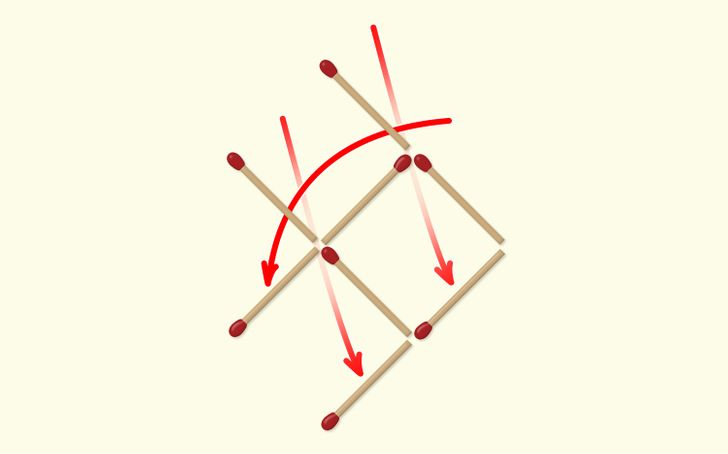## 9. Remove 3 matches so that you leave 3 squares.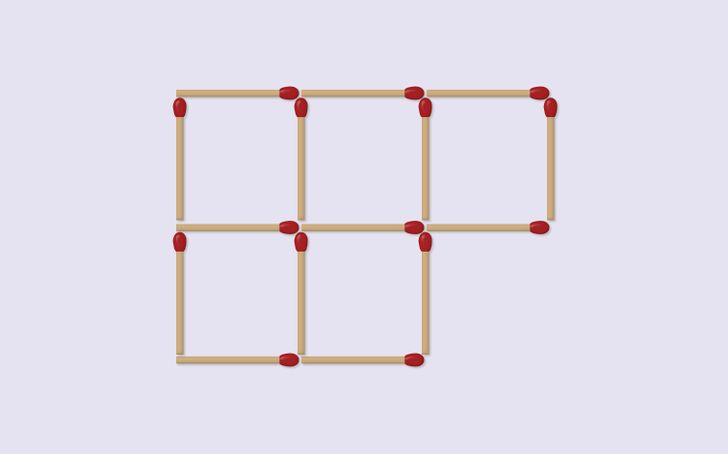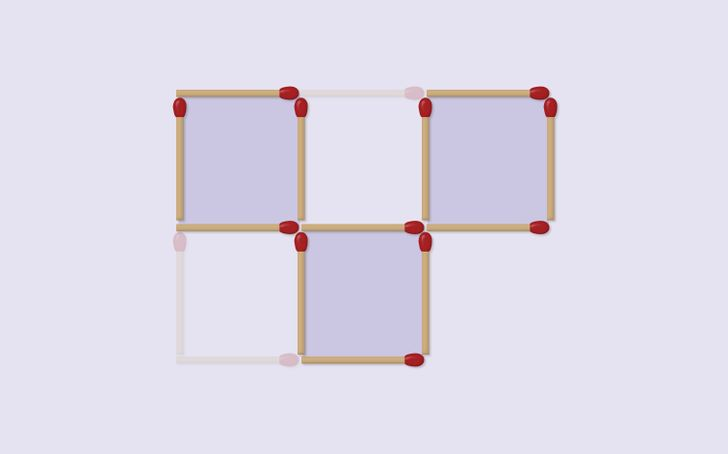## 10. Move 2 matches only to form 2 squares.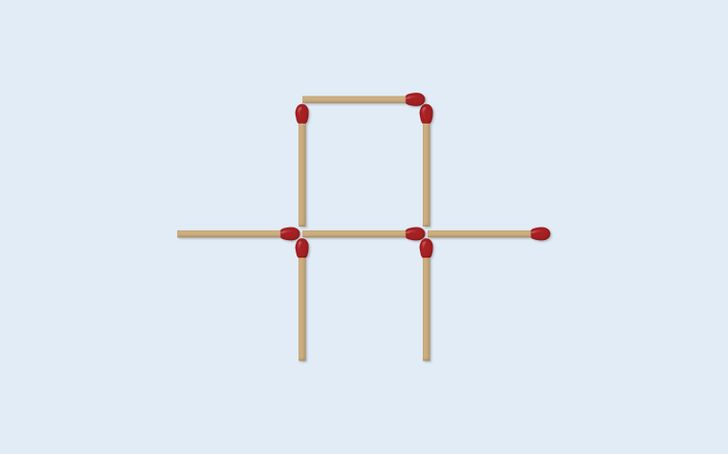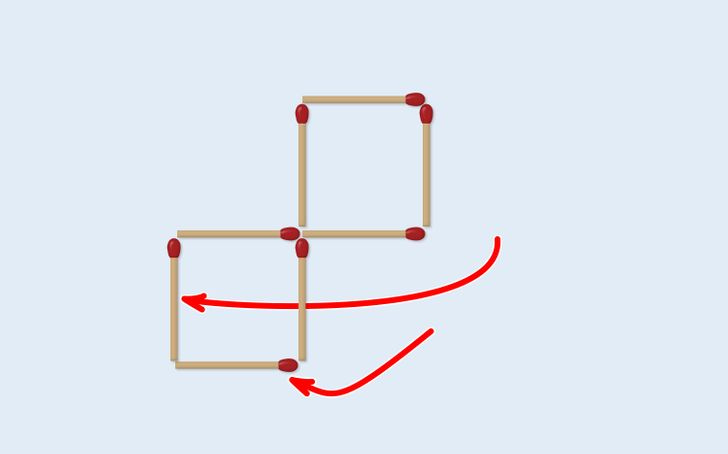## 11. Move only one match stick to form 4 triangles.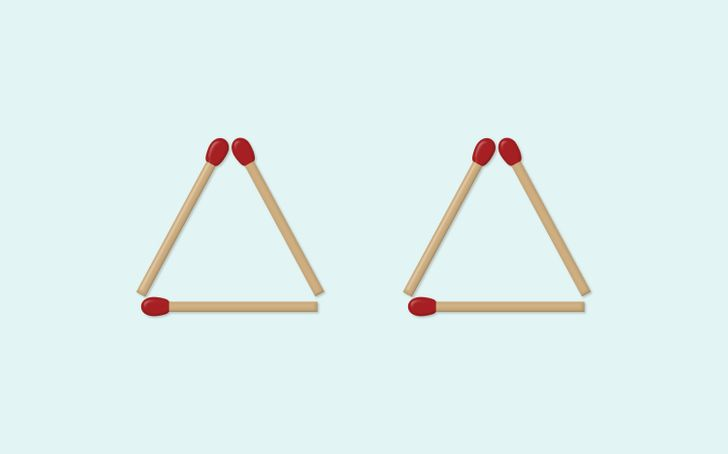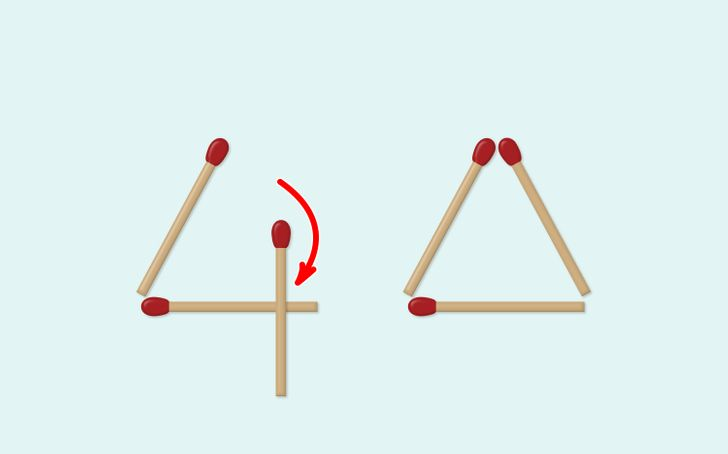## 12. Move 2 sticks only to form 3 triangles.## 13. Move only 2 matchsticks so you show the time 4:30.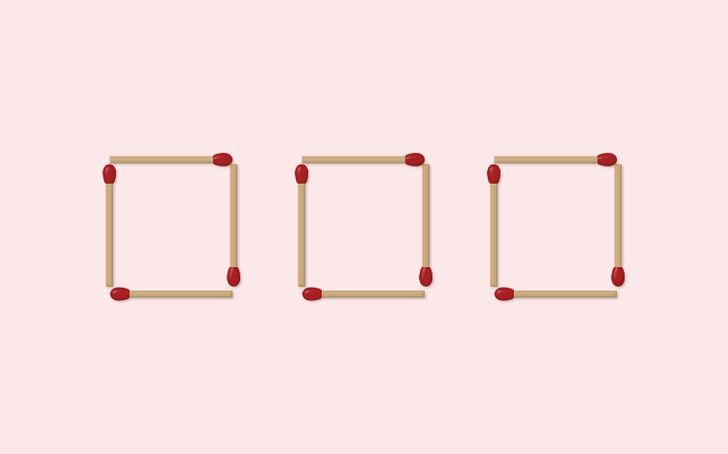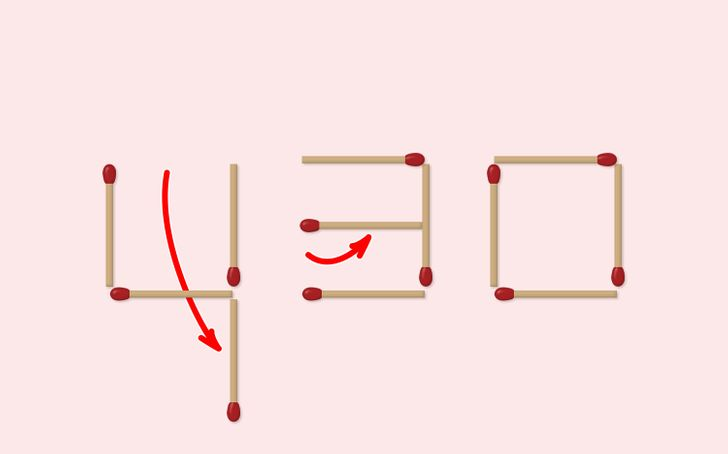## 14. Move 2 matchsticks to form 3 triangles.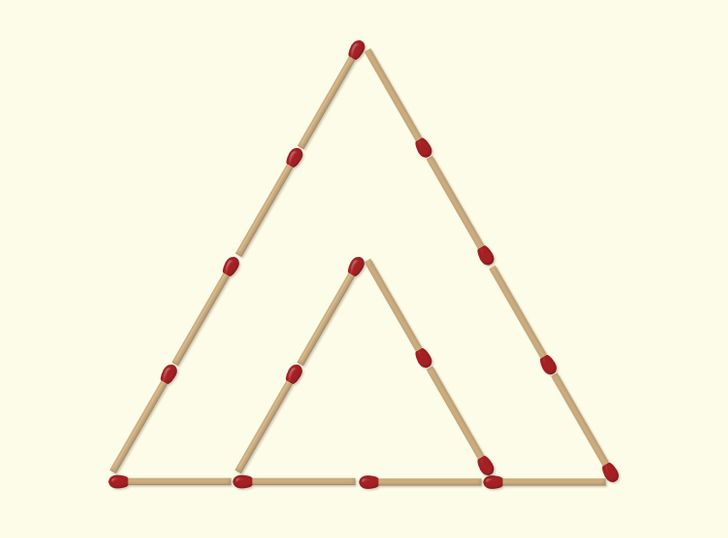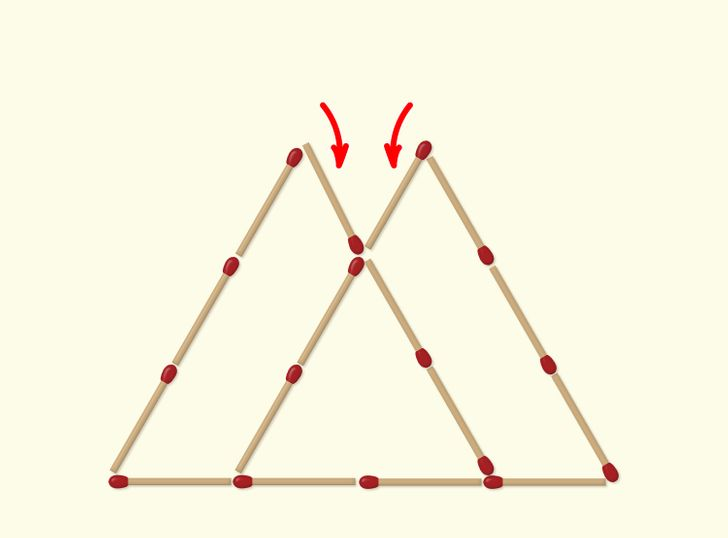## 15. Move 4 matches to form 3 squares.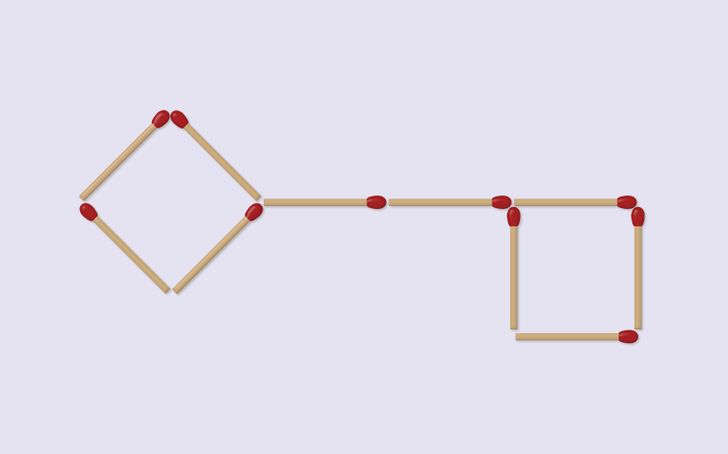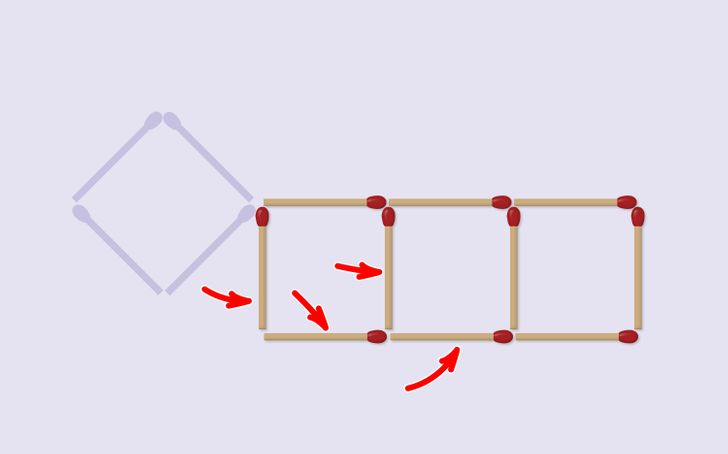## 16. Move 2 matchsticks so that you form 11 squares.